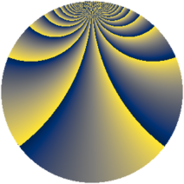# Properties

 Label 2240.2.bbLevel $2240$ Weight $2$ Character orbit 2240.bb Rep. character $\chi_{2240}(1009,\cdot)$ Character field $\Q(\zeta_{4})$ Dimension $144$ Sturm bound $768$

# Related objects

## Defining parameters

 Level: $$N$$ $$=$$ $$2240 = 2^{6} \cdot 5 \cdot 7$$ Weight: $$k$$ $$=$$ $$2$$ Character orbit: $$[\chi]$$ $$=$$ 2240.bb (of order $$4$$ and degree $$2$$) Character conductor: $$\operatorname{cond}(\chi)$$ $$=$$ $$80$$ Character field: $$\Q(i)$$ Sturm bound: $$768$$

## Dimensions

The following table gives the dimensions of various subspaces of $$M_{2}(2240, [\chi])$$.

Total New Old
Modular forms 800 144 656
Cusp forms 736 144 592
Eisenstein series 64 0 64

## Trace form

 $$144q + O(q^{10})$$ $$144q - 16q^{19} + 144q^{49} - 16q^{51} + 32q^{61} + 16q^{65} - 32q^{69} + 88q^{75} + 112q^{79} - 144q^{81} + 64q^{99} + O(q^{100})$$

## Decomposition of $$S_{2}^{\mathrm{new}}(2240, [\chi])$$ into newform subspaces

The newforms in this space have not yet been added to the LMFDB.

## Decomposition of $$S_{2}^{\mathrm{old}}(2240, [\chi])$$ into lower level spaces

$$S_{2}^{\mathrm{old}}(2240, [\chi]) \cong$$ $$S_{2}^{\mathrm{new}}(80, [\chi])$$$$^{\oplus 6}$$$$\oplus$$$$S_{2}^{\mathrm{new}}(320, [\chi])$$$$^{\oplus 2}$$$$\oplus$$$$S_{2}^{\mathrm{new}}(560, [\chi])$$$$^{\oplus 3}$$